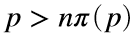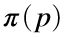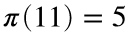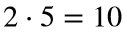# Problem 46594. Create a sequence of primes p such that p > n π(p)

Write a function to find the nth term of a sequence of prime numbers p such that, whereis the prime counting function. The first three numbers in this sequence are 2, 11, and 37. For example, the second number is 11 because five primes are less than or equal to 11 (i.e.,) and 11 >.
A related problem is Cody Problem 46117 Test approximations of the prime counting function.

### Solution Stats

54.55% Correct | 45.45% Incorrect
Last Solution submitted on Mar 31, 2023

### Community Treasure Hunt

Find the treasures in MATLAB Central and discover how the community can help you!

Start Hunting!